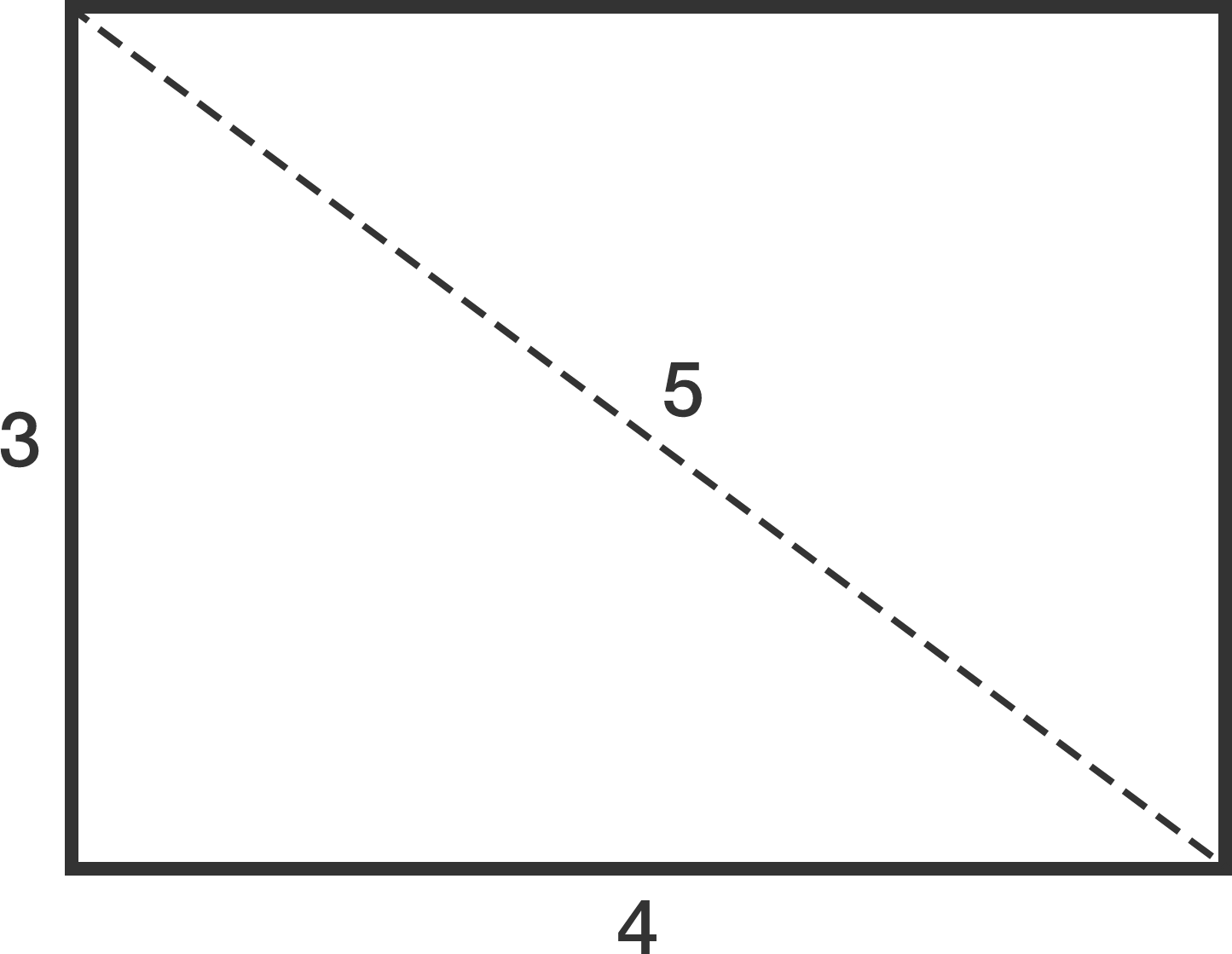# Is parametrization necessary?I want to construct a rectangle such that

• it has integer dimensions $a \times b$ that differ by exactly 1 $(\text{i.e. }|a-b|=1);$
• its diagonal also has an integer length.

The diagram to the right shows such a rectangle.

Is there any other way to construct a rectangle satisfying the above properties?

×